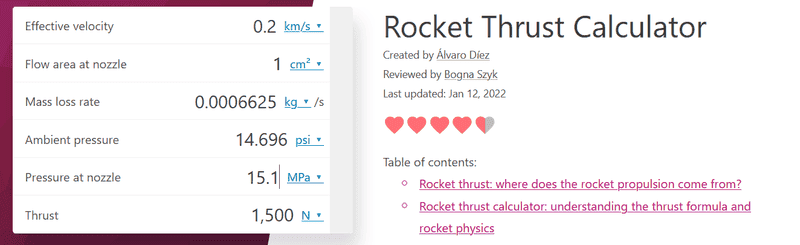# What's wrong with this math: A lot of thrust and almost no fuel?

• gggnano

#### gggnano

TL;DR Summary
Using basic calculator it seems as if a rocket can produce a lot of thrust and use little fuel if you increase pressure within practically possible range:10-20MPA?So according to this if you need a force that can lift a human and even an extremely ultra-light craft (1500 N) and have a velocity of 200 m/s you can eject extremely small amount of fuel through the nozzle: 0.0007 or less kg per second for as long as you're using 15 MPa pressure which is doable and even storage cylinders on alibaba use it. Another calculator shows that hydrogen stored at the same pressure will have just 18.5 kg weight per cubic meter? So this gives over 7 hours flight time with 720km/h??

Several things I don't understand: is 1cm^2 nozzle with 15.1 Mpa pressure possible? How is the effective velocity calculated especially if you aren't combusting but just open cylinder to release pressure? Why is the mass loss rate negative if you increase pressure? I should've solved this as a polynomial calculation since the calculator gives odd values unless you reload. Thank you.

The tool is assuming combustion, not a high pressure reservoir so your assumptions about what things mean is off.

^ Yeah...that's what I was suspecting...

[A trolling follow-up post by the OP has been deleted, and this thread will now be closed]

•topsquark